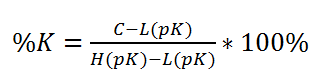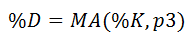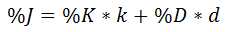## What is the kdj indicator?

One of the first predictors of a trend change is the KDJ Indicator. The KDJ indicator is a technical indicator used by Technical Analysts to analyze and predict changes in stock trends and price patters of a particularly traded asset.

The KDJ indicator is also known as the random index and is most commonly used in analyzing the market trend of a short-term position in a stock.

The KDJ is derived from the Stochastic Oscillator Indicator with only one difference being the addition of the “J” Line. The KDJ line has 3 values. %K, %D and %J.

The values of %K and %D lines show when a security is overbought or oversold. Overbought on a KDJ Indicator is above 80% and oversold is below 20%. The movements of the %K crossing %D are the moments for selling or buying. The J line signifies the divergence of the %D and the %K. The value of J can go below 0 and above 100 for the %K and %D lines on the chart.

## How to use the KDJ Indicator

Important Note: When the J Line crosses the %D and %K line it is usually signifying a change in the direction of the trend.When the %K line crosses in an upward pattern through the %D line it signifies the time to buy. This is usually in conjunction with the %J line moving in the same upward trend crossing the %K and %D line from below.

### Sell Signal

When the %K line crosses in a downward pattern through the %D line it signifies the time to sell. This is usually in conjunction with the %J line moving in the same downward trend crossing the %K and %D line from above.

A good thing to remember is that seasoned traders using the KDJ Indicator tend to wait till all three lines, %D, %K and %J to be either above the overbought (80%) zone before selling or under the oversold zone (20%) before buying. This is especially true if the J line is above %D and %K lines in the overbought zone and if the J line is under the %D and %K lines in the oversold zone.

Lastly, as with all other indicators, it is a good idea to support your signals with another indicator. When utilizing the KDJ Indicator, you could receive false signals. Adding an RSI (relative strength index) indicator can help to solidify the signals you receive from the KDJ Indicator.

## The Math - How is the kdj calculated?

For those of you interested in how the KDJ Indicator is calculated here is the math. The KDJ is calculated very similarly to the Stochastic Indicator however, as discussed, has the difference of the J Line being added which isn’t found on the Stochastic Indicator.

1. To find %K, we use the following equation:2. To create the %D line, use the following equation:3. Lastly, we find the %J line with this formula: